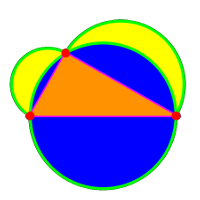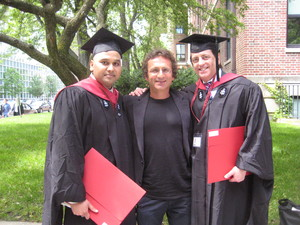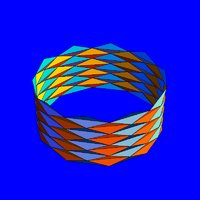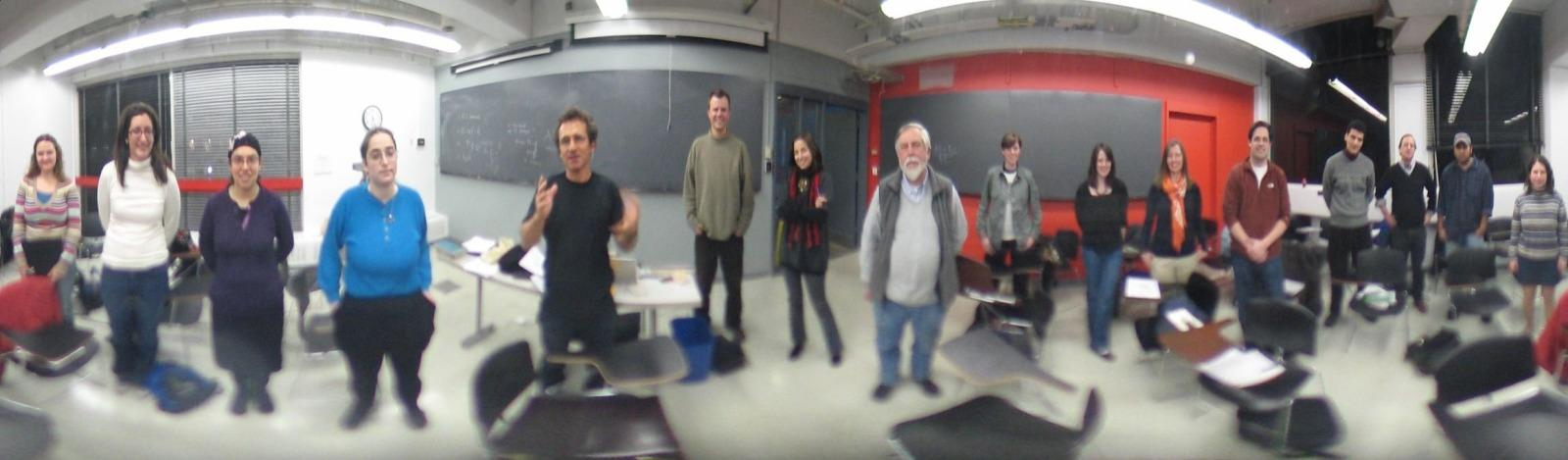Math E-320:
Teaching Math with a Historical Perspective
Mathematics E-320:
Instructor: Oliver Knill
Office: SciCtr 434

# From the teaching front

Added September 21, 2010: One of the teachers who took the class has used Minkowski theory in her own lesson and reported that the lesson went well. The students turned out to be inquisitive and discovered by themselves that there are symmetrical convex figures with area greater than four not intersecting the lattice at all if the figure is not centered on the origin. They asked: "Why did Minkowski center the figure on the origin?"

Such questions are hard to answer because the obvious answer "without that assumption there is no theorem" sounds cheap. It also provokes questions like whether there is a related theorem (with additional assumption) in which only the symmetry assumption with respect to an other point is assumed.Added June 2, 2010: graduation of Kalyan Mekala and Anselm Snodgrass (both students in Math E-320). Click for a larger photo.

# Dynamics

While working on the Collatz problem, Amselm brought up the Palyndrome 196 problem. Let S(x) be the number x written reversed. Like S(1262) = 2621. Define T(x) = x+S(x). A number x is called a Lychrel Number, if the orbit xn of x never hits the palindrome set P = { x = S(x) } . The following Mathematica code (written during class) does it
```F[n_]:=Module[{},s=IntegerDigits[n];m=Sum[s[[k]]10^(k-1),{k,Length[s]}];If[m==n,Print[n]];m+n]
ListPlot[Log[NestList[F,696,1000]]]
```
See p196.org.

Apropos Collatz: see this.

# Calculus

Nick sent this link about Ed Burger (who appeared in the presentation) winning an award for great teaching.

When looking at Poincaré's counter intuitive examples
```   sum  1000n/n!                 sum   n!/1000n
```
(the first sum diverges experimentally and the second one converges experimentally), we have all seen pretty quickly that the left hand side is just e1000 and convergent. To see that the right hand side is divergent, we had to improvise a bit but we found out with the help of Sterlings formula that already for n=3000, the terms n!/1000n are larger than 1.

Here are some additional and still sketchy remarks [PDF] complementing the summary handout.

Kalyan mentioned the Indian mathematician Madhava who has developed some series in the 14th century.

Added, April 3rd: Take it to the limit. A nice presentation of Archimedes argument on how to derive the area of a disc. First get the circumference, then the Area.Note that pictures can be misleading as the Schwarz paradox of 1890 shows and which I learned as a first year calculus student in Christian Blatter's book "Analysis I,II". one can triangulate a cylinder surface S of radius 1 and height into finer and finer pieces and take the limit of these areas and get infinity. Make a subdivision into n4 squares by subdividing the height into n3 and the angles into n pieces. We get a triangulation into 4 n4 triangles if we draw the diagonals in each square. The area of each triangle is large than 2 sin(pi/n) sin^2 (pi/2n) > 4/n3. The sum of the areas goes to infinity like n. Added, April 18, 2010: Strogatz column in the NYT on the fundamental theorem of calculus.

# Group Theory

Many puzzles are too difficult to cover in one hour. But there are some which work. The pyramorphix puzzle has 24 group elements. An other group accessible is part of the edge corner group of the rubik cube, which has only 36 elements. One can easily check this with GAP.
```gap> Size(Group(( 1,2,3,4)(5,6), ( 1,2)(3,4,5,6)));
36
```
Here is the size computation of the Rubik cube:
```rubik := Group(
( 1, 3, 8, 6)( 2, 5, 7, 4)( 9,33,25,17)(10,34,26,18)(11,35,27,19),
( 9,11,16,14)(10,13,15,12)( 1,17,41,40)( 4,20,44,37)( 6,22,46,35),
(17,19,24,22)(18,21,23,20)( 6,25,43,16)( 7,28,42,13)( 8,30,41,11),
(25,27,32,30)(26,29,31,28)( 3,38,43,19)( 5,36,45,21)( 8,33,48,24),
(33,35,40,38)(34,37,39,36)( 3, 9,46,32)( 2,12,47,29)( 1,14,48,27),
(41,43,48,46)(42,45,47,44)(14,22,30,38)(15,23,31,39)(16,24,32,40)
);
Size(rubik);

43252003274489856000
```
Online solver for rubic puzzles. Added March 8: An article of Melanie Bayley in the New York times takes about the Math in "Alice in Wonderland". Some quotes:

 In the mid-19th century, mathematics was rapidly blossoming into what it is today: a finely honed language for describing the conceptual relations between things. Dodgson found the radical new math illogical and lacking in intellectual rigor. In "Alice," he attacked some of the new ideas as nonsense - using a technique familiar from Euclid's proofs, reductio ad absurdum, where the validity of an idea is tested by taking its premises to their logical extreme. Early in the story, for instance, Alice's exchange with the Caterpillar parodies the first purely symbolic system of algebra, proposed in the mid-19th century by Augustus De Morgan, a London math professor. De Morgan had proposed a more modern approach to algebra, which held that any procedure was valid as long as it followed an internal logic. This allowed for results like the square root of a negative number, which even De Morgan himself called "unintelligible" and "absurd" (because all numbers when squared give positive results). The word "algebra," De Morgan said in one of his footnotes, comes from an Arabic phrase he transliterated as "al jebr e al mokabala," meaning restoration and reduction. He explained that even though algebra had been reduced to a seemingly absurd but logical set of operations, eventually some sort of meaning would be restored.

# Forth lecture

There are two difficulties to understand fully the proof of Wilson's theorem:

1) If p is a prime, then for any a, there is a pair b, such that a*b = 1 mod p has exactly one solution
2) If p is a prime, then the equation x2=1 mod p has two solutions x=1 and x=-1.
To 1) One could see this with Fermat's theorem: just take b = ap-2 to get one solution. It can not be that we have two solutions b,c because a*b = 1 mod p and a*c = 1 mod p implies a(b-c) = 0 mod p which means that p either divides a or (b-c). It can not divide a because a is smaller than p. It must divide (b-c) therefore, which means that b and c differ by a multiple of p.
To 2) x=1 and x=-1 both are solutions. Assume there is an other solution y, then y2-1 is divisible by p. Write y2 -1 = (y-1) (y+1). Therefore, p divides (y-1) or (y+1) which means that y differs from 1 by a multiple of p or differs from -1 by a multiple of p.

As Brahim has pointed out, it is not obvious to see from the expression
```      / n \     n (n-1) (n-2) .... (n-m+1)
(     ) = ---------------------------
\ m /      m (m-1) (m-2) ... 2 1
```
is an integer. It follows from the fact that these terms appear when expanding (x+1)n and from combinatorial consideration as the number of possibilities to chose m elements from n.

We have seen that this Binomial coefficient is divisible by n, if n is prime and if m is positive and smaller than m because there is nothing in the denominator which could cancel n.

It is actually true that all these terms are all divisible by n if and only if n is prime.

During the presentation part, I mentioned that the coincidence that the perfect number 28 is the length of the month might have contributed to the mystical attraction to primes. Eliza has pointed out that the month is not 28 days but 29. Indeed, there are many different months: on a lighter note, we can add that the average of the month lengths {27.554549878, 27.321661547, 27.321582241, 27.212220817, 29.530588853 } is 27.7881, pretty close to 28 .... Aliza also pointed out that the bible reference (given in Dickson) to the perfect number 6 is in the source actually referring to 5-6. While this confirms how crazy any numerology is, it is interesting to put one-selves into the mind of the spiritual past. It has influenced, how mathematics has developed thousands of years ago and why certain questions were persued more vigorously. Spiritual considerations certainly did influence the Pythagoreans.

Added February 24: we have seen in class how many open problems there are in number theory. Elementary number theory is attractive for amateur mathematicians because one can do so many experiments. As an example, I go an email tonight from a number theory enthusiast Bill McEachen about a conjecture he calls the primorial conjecture which is of a similar spirit than Goldbach. It is a claim about the primorial numbers pm(n) = p_1 p_2 ... p_n, the products of the first primes. The primorial numbers PM={2,6,30,210,...} grow much faster than the factorials {1,2,6,24,120,...}. If P={2,3,5,7,...} is the set of primes and Q={...-7,-5,-3,-2,-1,1,2,3,5,7,...}, the claim is that PM+Q contains all prime numbers. It is hard to get a grip on this numerically because primorials grow so fast. (I myself do not know much about this conjecture and only checked it for the first few thousand primes). Goldbach's claim is that P+P contains (2N+2), if N={1,2,3,4,...} is the set of natural numbers. The difficulty of these problems is that the addition and multiplication in N are so incompatible. In general, it is hard to make statements about the addition A+B={a+b | a in A, b in B} of sets. The Sieve of Erasthostenes with which our lecture started, is the statement that the complement of M*M is P, where M={2,3,4,5,6,...} and A*B = { a*b |a in A and b in B }. The complement of the primes P is a union of regular arithmetic progressions like 7M. But P is very irregular. Erastothenes insight is to note that M*M = (2M v 3M v 4M v ...) where v is the union and to see that this is an effective method to compute the primes.

# Third lecture

As expected, we did not have time for all handout topics, but we did work out Pythagoras, Thales and Hypochrates in detailed and discussed in the presentation part the 4 special points in the triangle. Some interesting points:
1. Hypocrates theorem can be refined: the areas of the "moons" can be related to areas of parts of the triangle. This remark of Francis is nice since it also needs some insight from Pythagoras proof not only from the result of Pythagoras.
2. As Eve has remarked, the proof of Thales theorem has to be done also in the case, when the point is such that the midpoint is not in the triangle.
3. It seems Anselm Snodgrass is right that Euclid did not state the believe that the 5th postulate is a consequence. At least its not obvious that he stated that. Certainly a few centuries later, mathematicians have raised the question of the independence, a question which culminated in obsession. It needed more than 1000 years more to settle it. Anselm also pointed out the book "Numbers and Infinity" of Sondheimer-Rogerson which is a quite nice reference in general. Anselm also showed me the passage from H.S.M. COxeters Non-Euclidean Geometry Sect 1.1 'Euclid showed the great strength of his genius by introducing Postulate, which is not self-evident like the others. (Moreover, his reluctance to introduce it provides a case for calling him the first non-Euclidean geometer!).'
4. After the class has found or seen the proofs of Pythagoras, Thales and Hypochrates (and Feuerbach only computer assisted), the class was divided about what is more "difficult": doing proofs or doing computations. I had asked, how come that highschool math often starts mathematics with proving theorems in geometry (a difficult task in my opinion) while for many college students, "proving things" is considered difficult and following concrete computations more comfortable.
Several great moments in mathematics have appeared today:
• Pythagoras theorem as the first result relating shape with numbers,
• Hypochrates theorem as the first result equating curvilinear shapes with linear shapes,
• Thales theorem as the first result of a nontrivial invariance appearing in geometry.
• Descartes bridge from algebra to geometry. We saw its power when running brute force but ice-cold proofs of all facts using Mathematica.
• The emergence of non Euclidean geometry.
• Riemann's building of new modern framework of geometry.
Added February 23, 2010: Cody Patterson told me about a nice proof that the altitudes of a triangle intersect: Add 2 equal and one flipped copy of the same triangle to relate the problem with the easier problem to get the intersection of perpendicular bisectors. See the exhibit.

Added March 15, 2010: The new Strogatz article in the NYT deals with geometry.

# Second lecture

We have seen two great moments in mathematics: the introduction of counting, and the realization that there are numbers beyond fractions. A few important things:
1. there are irrational numbers. This was the Pythagorean crisis.
2. We can approximate every irrational number by rational numbers. We have done that using Minkowski
3. it is crucial to have a good notation for numbers. We have seen that some notations are awkward, like the Greek or Roman system.
4. Quests to tune the number system went on until today. Surreal numbers were only introduced a few decades ago.
5. There are many open questions about irrational numbers. We do not know for example whether aa is irrational if a is a square root of 2.The class of today.
The New York times starts a series of commentaries by Steven Strogatz on mathematics. It fits this course well. The lecture of today works well with the first piece.

Here are some additional remarks about arithmetics [PDF] complementing the summary handout. Added February 14: Strogatz column about negative numbers explains why (-1) x (-1) = 1. Already the title of the column does. But the article explains well that such computations are on a different level than 3*4 = 12 which have a concrete realization as taking 3 groups of 4 objects. The computations for negative numbers follow rules so that an algebraic structure prevails and for example the arithmetic progression 2*(-1) = -2, 1*(-1) = -1, 0*(-1) = 0, (-1) * (-1) = 1 survives. The multiplication 3*(-4) = -12 still has an interpretation like 3 times borrowing 4 objects but (-4)x3 does not have it so easy any more. Today, Mathematicians rely on algebraic structures like "any Abelian group is also a Z module" and feel at home, but - as Strogatz points out - there can still be discomfort when learning or teaching how to work with negative numbers. One of the readers of the columns recalls a joke illustrating the mystery of negative numbers:
```A mathematician, a biologist and a physicist are sitting in a street cafe watching people going in and
coming out of the house on the other side of the street. First they see two people going into the house.
Time passes. After a while they notice three persons coming out of the house.
The physicist: "The measurement wasn't accurate."
The biologist: "They have reproduced."
The mathematician: "If now exactly one person enters the house then it will be empty again."
```
A math teacher explains it as follows:
```When good things happen to good people, it's good. (+)(+)=(+)
```
Added March 4, 2010: The earliest writings and maybe also computations seem older than thought. Eggshells used as containers were labeled (with numbers telling how much they contained?)

Added April 22, 2010: the following story shows that fractions can be tricky. Without a calculator it is obvious that 136 votes against 70 votes is less than 2/3 because 140/70 would be a 2/3 vote. I think the sin to use 0.66 for 2/3 is a typical spreadsheet sin. Using inaccurate numbers in spreadsheets combined with rounding errors make spreadsheet errors so abundant.

# First lecture

• As Troy has discovered, there are actually two lattice points and so in total three.
• While experimenting, we realized that it is helpful to try first with simple regions like triangles. It would be nice to work with deformable regions like wires. bu coming up with counter examples can take time.
• The main difficulty while presenting the proof of Minkowski's theorem the first time was to explain two two corresponding points which are mapped to the same point in the middle 2x2 square have coordinates A,B which satisfy A-B = (2n,2m) for some integers n,m. The exhibit tries to improve it.
• To the terminology in the handout: The word "lattice point" had not been explained well enough at first. It is a point (x,y) where both x and y are integers.
Added February 1, 2010: The article of Steven Strogatz in the New York Times mentioned below in the second lecture links to a nice article The unreasonable Effectiveness of mathematics in the natural sciences which fits well with the slide show. Wigner tries to give an answer to the question "What is Mathematics". For my lecture, I took a shortcut and just put "Mathematics is the science of structure". I personally find Wigner suggestion "Mathematics is the science of skillful operations with concepts and rules invented just for this purpose." as rather limiting. Operations do not necessarily have to be skillful and the operations do not necessarily have to be invented just for this purpose. I do not even believe that the operations have to be "invented". Mathematical operations exist and are used by nature without human invention. Mathematical truth would exist also without "skillful" humans to "invent" them.

Added February 23, 2010: the Notices of March 2010 contain an interview with Mikhail Gromov. He says: "Mathematics not only deals with what you see with your eye but what you see in the structure of things, at a more fundamental level, I would say." An other citation is by David Ruelle writes in his book "The Mathematician's Brain": "From what we have seen, mathematics appears to have a dual nature. On the one hand, it can be developed using a formal language, strict rules of deduction and a system or axioms. All the theorems can then be obtained and checked mechanically. We may call this the formal aspect of mathematics. On the other hand, the practice of mathematics is based on ideas, like Klein's idea of different geometries. This may be called the conceptual or structural aspect." These thoughts fit well with the definition attempt "Mathematics is the science of structure" given at the beginning of this course.